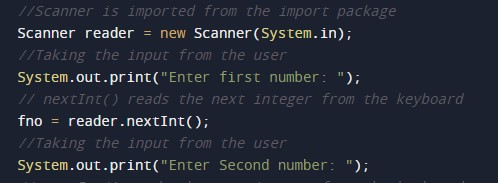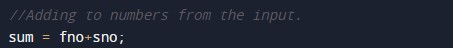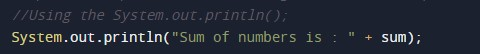# Java Program to Add Two Numbers

In this tutorial you will learn about the Java Program to Add Two Numbers and its application with practical example.

## Java Program to Add Two Numbers

In this tutorial, we will learn to create a Java program that will Add Two Numbers in Java programming.

## Prerequisites

Before starting with this tutorial, we assume that you are the best aware of the following Java programming topics:

• Operators in Java Programming.
• Basic Input and Output function in Java Programming.
• Basic Java Programming.

The addition in mathematics is the sum of two numbers. The arithmetic operations are done using the arithmetic operators. These operators are used between two arithmetic operands or between two numbers.

## Program to Add Two Numbers:-

In this tutorial, we will create a program that will add the two numbers using an addition operator. We will first take two numbers in input, and then we will add them and store the value in the third variable.

As we all know, the integer, float, or double data types in java. In programming, we can take the input from the user in numbers format. In today’s program, we will take input in number format. Secondly, we will add the number with the help of a small program. The numbers are the most useful terminology in any programming language. For number/arithmetic manipulation, there are many predefined functions available.

With the help of this program, we can take input and Add Two Numbers.

## Output:-In the above program, we have first initialized the required variable.• fno = it will hold the input number value from the user.
• sno = it will hold the input number value from the user.
• sum = it will hold the sum value of the numbers.

Taking input numbers from the user.Calculating the sum of two numbers.Printing the output sum of two numbers.In this tutorial we have learn about the Java Program to Add Two Numbers and its application with practical example. I hope you will like this tutorial.